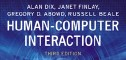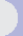HCI book > exercises > 17. system models > ex. 17.7 text onlyexercises17. models of the systemEXERCISE 17.7

Sections 17.2.1 and 17.2.2 give two reasons for using formal methods in HCI: communication and analysis. These are focused on the sort of mathematical models found in chapter 17. However, there are other sort of 'formal' modelling in HCI: dialog notations are formal models of the syntax of the human­computer conversation, hierarchical task analysis is a formalisation of the task structure, some cognitive models are effectively formal models of the user's mind.

• Are communication and analysis reasons for using these other sorts of formalism?
• Can you think of other reasons why you would or would not use formalisms?
• Try to use these pros and cons to formulate issues in the choice of appropriate forms of formal model and analysis.

answer available for tutors only

Very similar arguments apply to dialog notations as to more mathematical notations. HTAs are certainly used for communication, but perhaps not so much for analysis. Cognitive models are aimed more towards analysis (e.g. learning complexity with TAG, timing with KLM).

• difficult to learn
• time consuming (and therefore costly)
• only understood by a few and hence not so good for communication

• can save time (see 'It works!' page 561 of Human-Computer Interaction)
• helps discover potential usability problems at design stage (see section 16.6)
• analysis can be automated (see box 'automatic analysis' on page 586)

Some issues in choosing an appropriate formalism

• Who is involved?
• Do they know any particular techniques?
• Will they repeatedly use the same techniques or is this a one-off need?
• How complex is the problem?
• Are there likely to be difficult issues that it is hard to solve using informal reasoning?
• How costly will mistakes be?
 Other exercises in this chapter ex.17.1 (ans), ex.17.2 (ans), ex.17.3 (ans), ex.17.4 (ans), ex.17.5 (tut), ex.17.6 (tut), ex.17.7 (tut), ex.17.8 (tut), ex.17.9 (tut), ex.17.10 (tut)all exercises for this chapter